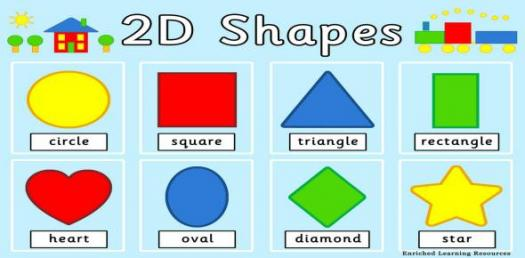# Properties Of Shape Quiz

10 Questions | Total Attempts: 324SettingsThe geometry of simple shapes is part of elementary mathematics. In simple geometry, we understand properties of several solid shapes like triangle, square, rectangle and many others. Each of these shapes have definite number of sides or vertices and angles. How much do you know about shapes? Try this quiz now!

Questions and Answers
• 1.
How many sides does a square have?
• A.

Three sides

• B.

Twelve sides

• C.

Two sides

• D.

Four sides

• 2.
What is the sum of angles in a triangle?
• A.

130 degrees

• B.

150 degrees

• C.

180 degrees

• D.

200 degrees

• 3.
How many angles are equal in an equilateral triangle?
• A.

Three angles

• B.

Two angles

• C.

Four angles

• D.

Nine angles

• 4.
How many vertices are in a quadrilateral?
• A.

Four vertices

• B.

Six vertices

• C.

Nine vertices

• D.

Ten vertices

• 5.
What is the sum of exterior angles of a polygon?
• A.

90 degrees

• B.

360 degrees

• C.

180 degrees

• D.

120 degrees

• 6.
Which of these is not a basic shape?
• A.

Square

• B.

Triangle

• C.

Rectangle

• D.

Decagon

• 7.
What is the line that divides a circle into two called?
• A.

Diameter

• B.

Radius

• C.

Segment

• D.

Tangent

• 8.
What is the sum of angles in a right-angle triangle?
• A.

270 degrees

• B.

90 degrees

• C.

120 degrees

• D.

360 degrees.

• 9.
How many sides are equal in an isosceles triangle?
• A.

Two sides

• B.

A side

• C.

Three sides

• D.

Four sides

• 10.
How many lines of symmetry are in an isoceles triangle?
• A.

Two lines of symmetry

• B.

Three lines of symmetry

• C.

Four lines of symmetry

• D.

A line of symmetry

Related Topics